# Boundary conditions

Boundary conditions (BC) are physical definitions on domain boundary surfaces for thermal conditions and electromagnetism. Here are listed all boundary conditions used in CENOS, as well as the most common usage for each BC.

## Thermal BC​

Thermal BC's are boundary conditions through which the heat exchange between domains is defined.

### Fixed temperature​

Fixed temperature boundary condition defines a fixed temperature on the boundary surface.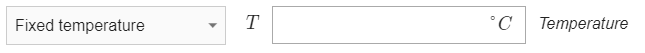usage

When Motion is used on infinite billet, the surface through which the billet enters the computational domain can be defined with Fixed Temperature.

### Heat flux​

Heat flux boundary condition defines a constant heat flux density on the boundary surface.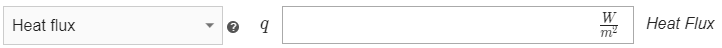usage

Can be used if the heat flux going through a specific area is known

### Heat flow​

Heat flow boundary condition defines a constant heat flow (in watts) on the boundary surface.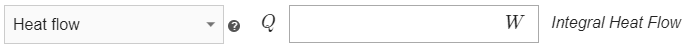usage

Can be used if the heat flow going through a specific area is known

### Convection​

Convection boundary condition defines the heat flux as dependent from the ambient temperature and heat transfer coefficient. Convection is defined as:

$q=h(T-T_{amb})$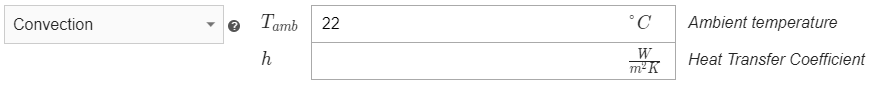usage

Convection is used on the workpiece-air interface to define heat exchange when temperature is low. Usually used together with Radiation.

Radiation boundary condition defines the thermal radiation to infinity on the boundary surface, where the heat flux is defined as:

$q=\sigma_{SB}\epsilon(T^4-T_{amb}^4)$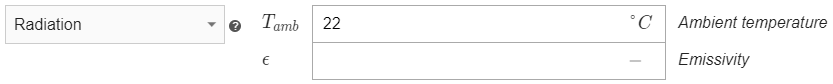usage

Radiation is used on the workpiece-air interface to define heat exchange when temperature is high. Usually used together with Convection.

### Combined​

Combined boundary condition allows you to combine multiple BC such as Convection, Radiation, Heat Flux and Heat Flow on a single domain surface.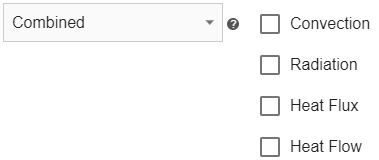usage

Combined is used on workpiece-air interface to define both Convection and Radiation for a complete heat trasfer definition over wide temperature range.

Adiabatic boundary condition defines 0 heat flux on the surface.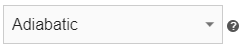usage

Adiabatic is used on symmetry surfaces for domains with thermal analysis.

### Interface​

Interface boundary condition defines boundary surface as the internal surface between two domains.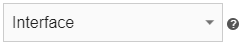usage

Used to define interface between two domains which both have thermal analysis definition.

### Thermal Contact Resistance​

Thermal Contact Resistance boundary condition defines thermal resistance between two domains.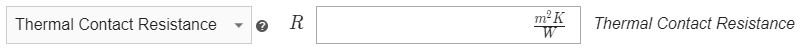usage

Used to define interface between two domains who both have thermal analysis definition.

## Electromagnetics​

Electromagnetic BC's are boundary conditions through which the magnetic flux is defined.

### Infinity​

Infinity boundary condition defines a zero vector potential on the boundary surface.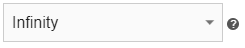usage

Infinity is used to define the outer surface of the air box.

### Flux parallel​

Flux parallel boundary condition defines the magnetic field lines as parallel to the boundary surface.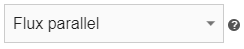usage

Flux parallel is used to define symmetry boundary conditions on the sides of slices and sectors.

### Flux normal​

Flux normal boundary condition defines the magnetic field lines as normal to the boundary surface.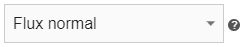usage

Flux normal is used to define symmetry boundary conditions on top and bottom of slices and sectors.

### Interface​

Interface boundary condition defines boundary surface as the internal surface between two domains.usage

Used to define interface between two domains from which only one has thermal analysis definition.

### Surface Impedance​

Surface Impedance boundary condition can be accessed only through the Surface Impedance domain. It defines the boundary surface as an infinitely thin layer for VH frequency skin layer resolution.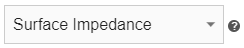usage

Surface Impedance is used to replace manual skin layer resolution through mesh at very high frequency setup.

### Symmetry axis​

Symmetry axis is a 2D-axial-symmetric specific boundary condition which defines 0 vector potential on the symmetry axis.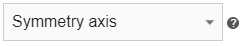usage

Symmetry axis is used in every 2D axial-symmetric case to define rotational axis.

## Current source​

Current BC's are accessed only under the Current source domain and through which the current or voltage flowing through the conductor are defined.

### Voltage​

Voltage boundary condition defines the voltage on the boundary surface.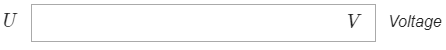usage

Voltage is used to define the voltage on one of inductor terminals. For the other terminal use Ground.

### Current​

Current boundary condition defines a positive current supply to the boundary surface and the value of current.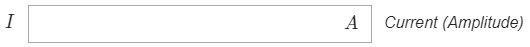usage

Current is used to define current on one of the inductor terminals. For the other terminal use Ground.

### Ground​

Ground boundary condition defines a negative current supply to the boundary surface.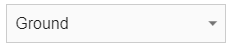usage

Ground is used to define one of the inductor terminals. For the other terminal use Voltage or Current.